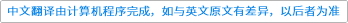Computer Communications ( IF 2.816 ) Pub Date : 2021-04-07 , DOI: 10.1016/j.comcom.2021.03.025
Mohamad Khattar Awad, Mohammed W. Baidas, Ahmad A. El-Amine

Joint transmission coordinated multi-point (JT-CoMP) and non-orthogonal multiple access (NOMA) are key enabling technologies of 5G ubiquitous broadband infrastructures. These technologies are jointly expected to exploit multi-cell and non-orthogonal resource transmissions; thus, conventional resource allocation schemes that only consider either one of them fail to efficiently exploit resources of 5G networks. In this paper, we bridge this gap by proposing a practical and comprehensive joint sub-carrier assignment and power allocation scheme for network sum-rate maximization in JT-CoMP-enabled NOMA networks. We formulate the problem as a mixed integer non-linear programming (MINLP) problem, which is NP-hard. The problem is decoupled into two sub-problems, where the sub-carrier assignment is modeled as a two-sided many-to-many matching game and the power allocation is formulated as a difference of convex (DC) programming problem. The matching algorithm is proved to converge to a two-sided exchange stable matching. Furthermore, the solution computed by the proposed scheme is verified against a baseline solution computed by a commercial optimization package, and has been shown to achieve 91.38% of the baseline solution for JT-CoMP-NOMA networks. Simulation results illustrate that the proposed scheme enhances cell-edge users’ achievable rates by $0.1-27.7\text{%}$ in JT-CoMP-NOMA over conventional NOMA.down
wechat
bug